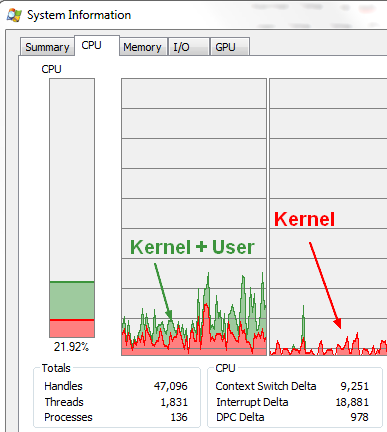# CPU - (CPU|Processor) Time Counter

Cpu time

The count of cycles, also known as clockticks, forms the basis for measuring how long a program takes to execute.

## Definition

How do you measure Execution Time?

$$\begin{array}{rll} && \text{Kernel} && \text{Time} \\ + &&\text{User} && \text{Time} \\ \hline \\ && \text{Total} && \text{Time} \end{array}$$

CPU Time Running which code
Kernel (Sys) Time Operating system code - the time of CPU spent on the kernel (system) code only within the given process - other processes and the time when our process is blocked are not included.
User Time Time CPU spends running the program code (ie CPU time spent on non-kernel (user-mode) code only within the given process – so other processes and the time when our process is blocked are not included.).
Total time User + Kernel
Real Time Wall clock (total elapsed time) (can be lower than total time because of parallelism or longer because of wait) - user-perceived time it took to execute the command – from the start to the end of the call, including time slices used by other processes and the time when our process is blocked (e.g. I/O waiting)

## Process Explorer

CPU Time in process explorer

 The performance tab of a process in Process Explorer. Red in the CPU usage graph indicates CPU usage in kernel-mode whereas green is the sum of kernel-mode and user-mode execution.A data collector set can be configured via logman.exe to log the “% Processor Time” counter in the “Processor Information” object for this purpose.

## Formula

Every conventional processor has a clock with a fixed cycle time (or clock rate). At every CPU cycle, an instruction is executed.

$$\text{CPU Time} = \text{CPU cycles executed} * \text{Cycle times}$$

$$\text{CPU cycles} = \text{Instructions executed} * \text{Average Clock Cycles per Instruction (CPI)}$$

Putting it all together: $$\text{CPU Execution Time} = \text{Instructions count} * \text{CPI} * \text{Clock Cycle Time}$$ where:

$$\begin{array}{rll} \text{Instructions Count} && = && \fracInstructions}Programs} \\ \text{CPI} && = && \fracCycles}Instruction} \\ \text{Clock Cycle Time} &&= && \fracSeconds}Cycle} \end{array}$$

Note:

• CPI is somewhat artificial (computed from the other numbers using) but it's seems to be intuitive and useful.
• Use dynamic instruction count (#instructions executed), not static (#instructions in compiled code)

## Performance

Response time distributed through the request lifetime

### Busy vs Wait

Percentage shown in monitoring tool is generally: $$\text{CPU Busy vs Wait Event} = \frac{\text{User} + \text{Kernel}}{\text{Wallclock Time}}$$

### CPU Performance

$$\text{CPU Performance} = \frac{1}{\text{Total CPU Time}}$$

### System Performance

$$\text{System Performance} = \frac{1}{\text{Wallclock Time}}$$

## Units (Mhz to ns)

• Rate often measured in MHz = millions of cycles/second
• Time often measured in ns (nanoseconds)

$$\begin{array}{rl} {X} \mbox{ MHz } && = && \frac1000}X} \mbox{ ns} \\ 500 \text{ MHz} && \approx && 2 \text{ ns clock} \end{array}$$

## Documentation / Reference

• the time linux utility.